# P-rank

(diff) ← Older revision | Latest revision (diff) | Newer revision → (diff)

(in group theory)

Let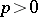be a prime number. The-rank of a finite groupis the largest integersuch thathas an elementary Abelian subgroup of order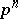(cf. Abelian group). A-group is elementary Abelian if it is a direct product of cyclic groups of order(cf. Cyclic group). A finite grouphas-rankif and only if either the Sylow-subgroup (cf. Sylow subgroup) ofis cyclic or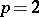and the Sylow-subgroup ofis generalized quarternion. There are several variations on the definition. For example, the normal-rank ofis the maximum of the-ranks of the Abelian normal subgroups of(cf. Normal subgroup). The sectional-rank ofis the maximum of the-ranks of the Abelian sections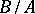for subgroups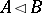of.

The notion of-rank was used extensively to sort out cases in the classification of finite simple groups (cf. Simple finite group). Some details can be found in [a2] and [a3]. In particular, see [a3], Sect. 1.5. In [a2], the word "p-depth of a groupdepth" is used and "rank" is reserved for a different concept. In the cohomology of groups, a celebrated theorem of D. Quillen [a4] states that the-rank ofis the same as the Krull dimension (cf. Dimension) of the modulocohomology ring of. The connection can be described as follows. Supposeis a field of characteristic. Letbe an elementary Abelian subgroup of order. By direct calculation it can be shown that the cohomology ring ofmodulo its radical is a polynomial ring invariables. Hence its maximal ideal spectrum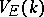is an affine space of dimension. Quillen's theorem says that the restriction mapping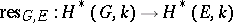induces a finite-to-one mapping of varieties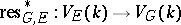and, moreover,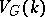is the union of the images for all. Therefore, the dimension of, which is the Krull dimension of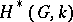, is the maximum of the-ranks of the subgroups. The theorem has found many applications in modular representation theory (see [a1]).

How to Cite This Entry:
P-rank. Encyclopedia of Mathematics. URL: http://encyclopediaofmath.org/index.php?title=P-rank&oldid=13520
This article was adapted from an original article by J. Carlson (originator), which appeared in Encyclopedia of Mathematics - ISBN 1402006098. See original article